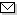Language:   Search:   Contact# Search and Browse the MSC 2000

Search:
Please note that in the second half of 2009, MSC2010 is deployed in the production of ZMATH and will become official standard in January 2010.

Enter a query and click »Search«...

34-XX Ordinary differential equations → ZMATH
34K05 General theory → ZMATH
34K06 Linear functional-differential equations → ZMATH
34K07 Theoretical approximation of solutions → ZMATH
34K10 Boundary value problems → ZMATH
34K11 Oscillation theory → ZMATH
34K12 Growth, boundedness, comparison of solutions → ZMATH
34K13 Periodic solutions → ZMATH
34K14 Almost periodic solutions → ZMATH
34K17 Transformation and reduction of equations and systems, normal forms → ZMATH
34K18 Bifurcation theory → ZMATH
34K19 Invariant manifolds → ZMATH
34K20 Stability theory → ZMATH
34K23 Complex (chaotic) behavior of solutions → ZMATH
34K25 Asymptotic theory → ZMATH
34K26 Singular perturbations → ZMATH
34K28 Numerical approximation of solutions → ZMATH
34K29 Inverse problems → ZMATH
34K30 Equations in abstract spaces [See also 34Gxx, 47Dxx, 47Jxx] → ZMATHAbel prize 2010I. M. Gelfand 1913-2009MSC2010